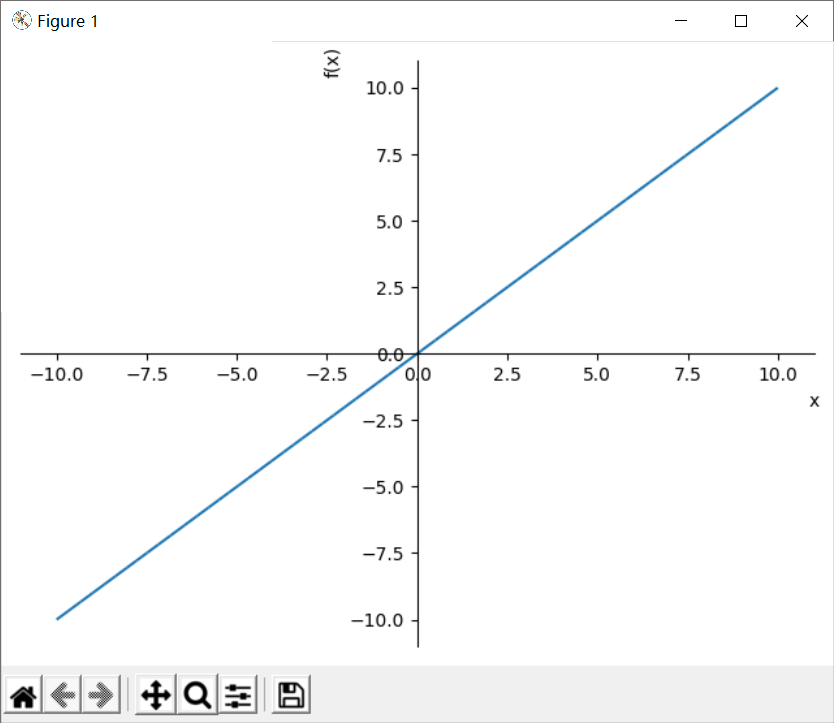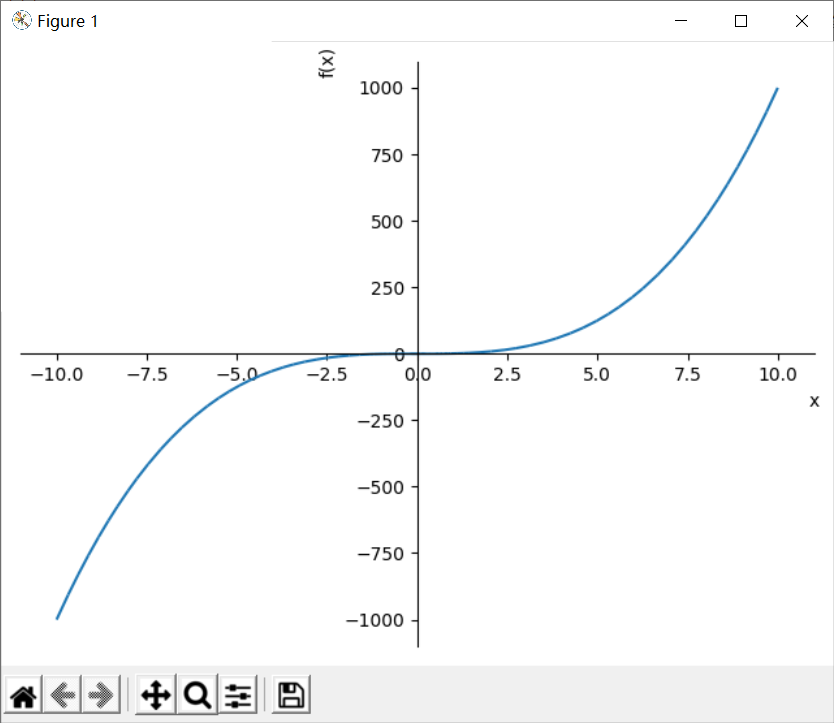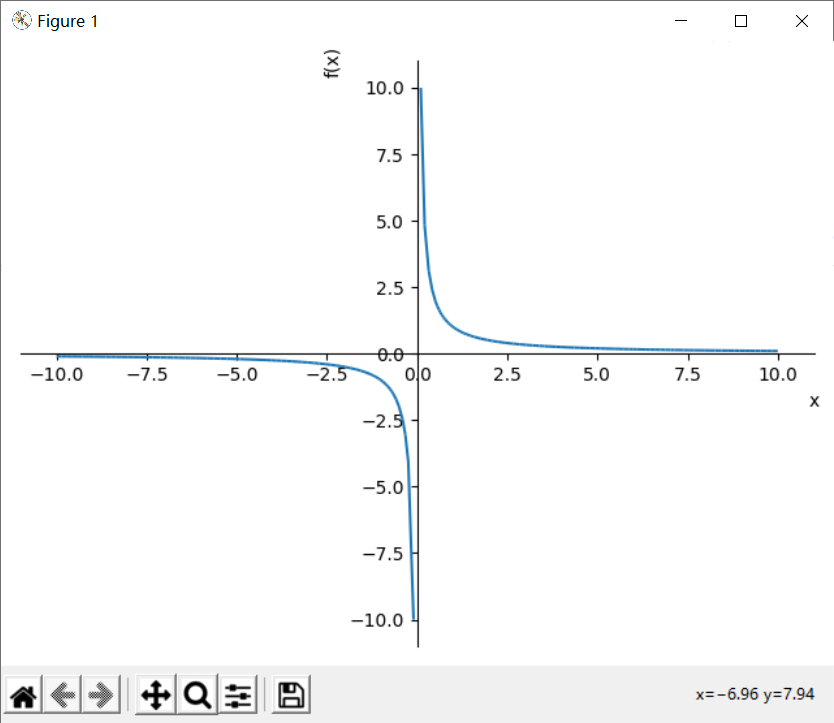1764字，阅读需时6分钟y = x^a

a是实数，函数的定义域要看a的取值而定。当a取任何实数时，函数在(0,+∞)区间内总有定义；当a>0时，函数在[0,+∞)区间内总有定义。

y = x，y=x^2，y=x^3，y=x^1/2，y=x^-1是最常见的幂函数，下面分别探讨它们的图像和性质。

```# 导入sympy库
from sympy import symbols,sin,plot
# 定义幂函数
def func(x,y):
return x**y
# 定义数学符号x,y
x=symbols('x')
y=symbols('y')
# 生成y=x函数公式
f1=func(x,1)
# 绘制图形
plot(f1,(x,-10,10))```

plot是绘制图形的函数，它可以传入多个函数公式，(x,-10,10)是一个元组，用于定义函数自变量的区间，这里定义了变量x的区间为[10,10]。f1=func(x,1)

f1=func(x,2)f1=func(x,1)

f1=func(x,3)f1=func(x,1)

f1=func(x,1/2)例5 y=x^-1的图像和性质

```# 导入sympy库
from sympy import symbols,sin,plot
# 定义幂函数
def func(x,y):
return x**y
# 定义数学符号x,y
x=symbols('x')
y=symbols('y')
# 生成y=x函数公式
f1=func(x,-1)
# 绘制图形
plot((f1,(x,0.1,10)),(f1,(x,-10,-0.1)))```